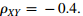Create an Account

Home / Questions / Two jointly Gaussian zero-mean random variables X and Y have respective variances of 3 and...

Two jointly Gaussian zero-mean random variables X and Y have respective variances of 3 and 4 and correlation coefficient A new random variable is defined as Z = X 2Y Write down an expression for

Two jointly Gaussian zero-mean random variables, X and Y, have respective variances of 3 and 4 and correlation coefficientA new random variable is defined as Z = X + 2Y. Write down an expression for the pdf of Z.

Jul 31 2020 View more View LessSubscribe To Get Solution